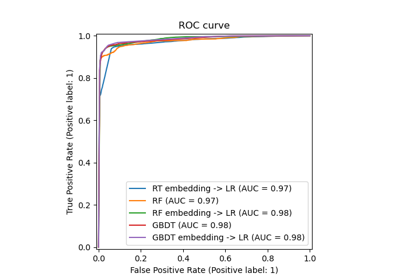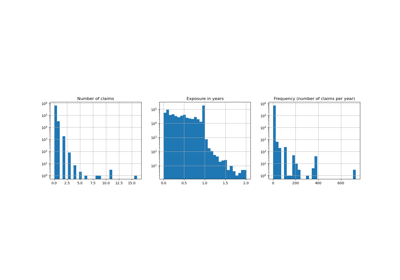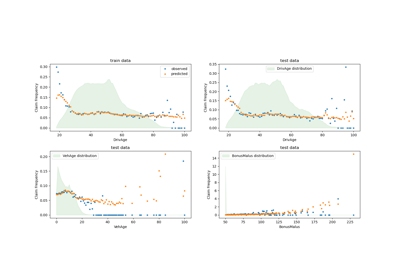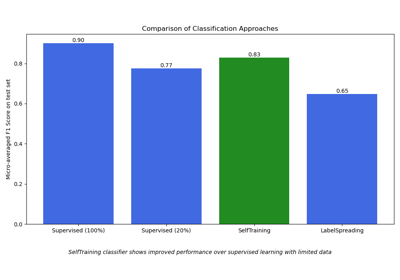# sklearn.preprocessing.FunctionTransformer¶

class sklearn.preprocessing.FunctionTransformer(func=None, inverse_func=None, *, validate=False, accept_sparse=False, check_inverse=True, kw_args=None, inv_kw_args=None)[source]

Constructs a transformer from an arbitrary callable.

A FunctionTransformer forwards its X (and optionally y) arguments to a user-defined function or function object and returns the result of this function. This is useful for stateless transformations such as taking the log of frequencies, doing custom scaling, etc.

Note: If a lambda is used as the function, then the resulting transformer will not be pickleable.

New in version 0.17.

Read more in the User Guide.

Parameters
funccallable, default=None

The callable to use for the transformation. This will be passed the same arguments as transform, with args and kwargs forwarded. If func is None, then func will be the identity function.

inverse_funccallable, default=None

The callable to use for the inverse transformation. This will be passed the same arguments as inverse transform, with args and kwargs forwarded. If inverse_func is None, then inverse_func will be the identity function.

validatebool, default=False

Indicate that the input X array should be checked before calling func. The possibilities are:

• If False, there is no input validation.

• If True, then X will be converted to a 2-dimensional NumPy array or sparse matrix. If the conversion is not possible an exception is raised.

Changed in version 0.22: The default of validate changed from True to False.

accept_sparsebool, default=False

Indicate that func accepts a sparse matrix as input. If validate is False, this has no effect. Otherwise, if accept_sparse is false, sparse matrix inputs will cause an exception to be raised.

check_inversebool, default=True

Whether to check that or func followed by inverse_func leads to the original inputs. It can be used for a sanity check, raising a warning when the condition is not fulfilled.

New in version 0.20.

kw_argsdict, default=None

Dictionary of additional keyword arguments to pass to func.

New in version 0.18.

inv_kw_argsdict, default=None

Dictionary of additional keyword arguments to pass to inverse_func.

New in version 0.18.

Examples

>>> import numpy as np
>>> from sklearn.preprocessing import FunctionTransformer
>>> transformer = FunctionTransformer(np.log1p)
>>> X = np.array([[0, 1], [2, 3]])
>>> transformer.transform(X)
array([[0.       , 0.6931...],
[1.0986..., 1.3862...]])


Methods

 fit(X[, y]) Fit transformer by checking X. fit_transform(X[, y]) Fit to data, then transform it. get_params([deep]) Get parameters for this estimator. Transform X using the inverse function. set_params(**params) Set the parameters of this estimator. Transform X using the forward function.
fit(X, y=None)[source]

Fit transformer by checking X.

If validate is True, X will be checked.

Parameters
Xarray-like, shape (n_samples, n_features)

Input array.

Returns
self
fit_transform(X, y=None, **fit_params)[source]

Fit to data, then transform it.

Fits transformer to X and y with optional parameters fit_params and returns a transformed version of X.

Parameters
Xarray-like of shape (n_samples, n_features)

Input samples.

yarray-like of shape (n_samples,) or (n_samples, n_outputs), default=None

Target values (None for unsupervised transformations).

**fit_paramsdict

Additional fit parameters.

Returns
X_newndarray array of shape (n_samples, n_features_new)

Transformed array.

get_params(deep=True)[source]

Get parameters for this estimator.

Parameters
deepbool, default=True

If True, will return the parameters for this estimator and contained subobjects that are estimators.

Returns
paramsdict

Parameter names mapped to their values.

inverse_transform(X)[source]

Transform X using the inverse function.

Parameters
Xarray-like, shape (n_samples, n_features)

Input array.

Returns
X_outarray-like, shape (n_samples, n_features)

Transformed input.

set_params(**params)[source]

Set the parameters of this estimator.

The method works on simple estimators as well as on nested objects (such as Pipeline). The latter have parameters of the form <component>__<parameter> so that it’s possible to update each component of a nested object.

Parameters
**paramsdict

Estimator parameters.

Returns
selfestimator instance

Estimator instance.

transform(X)[source]

Transform X using the forward function.

Parameters
Xarray-like, shape (n_samples, n_features)

Input array.

Returns
X_outarray-like, shape (n_samples, n_features)

Transformed input.

## Examples using sklearn.preprocessing.FunctionTransformer¶Feature transformations with ensembles of trees¶Poisson regression and non-normal loss¶Tweedie regression on insurance claims¶Column Transformer with Heterogeneous Data Sources¶Semi-supervised Classification on a Text Dataset¶